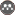Volume 8, Issue 3 (fall 2004)                   JWSS 2004, 8(3): 1-13 | Back to browse issues page

BibTeX | RIS | EndNote | Medlars | ProCite | Reference Manager | RefWorks
Send citation to:H. R. Fooladmand, A. R. Sepaskhah, J. Niazi. Estimating Soil- Moisture Characteristic Curve Based on Soil Particle Size Distribution Curve and Bulk Density. JWSS. 2004; 8 (3) :1-13
URL: http://jstnar.iut.ac.ir/article-1-317-en.html
Abstract:   (29014 Views)
To obtain soil-moisture characteristic curve experimentally is time-consuming and usually subject to considerable errors. So, many investigators have tried to predict soil-moisture characteristic curve by different models. One of these models predicts soil moisture characteristic curve based on soil particle size distribution and bulk density. In this model, soil particle size distribution curve is divided into a number of segments, each with a specific particle radius and cumulative particle mass greater than that of the radius. Using these data, soil-moisture characteristic curve was estimated. In this model, a scale factor, α, is used which may be considered as a constant, or obtained by logistic or linear procedures. The average values of α for clay, silty clay, sandy loam, two loam soils, and two silty clay loam soils were 1.159, 1.229, 1.494, 1.391, 1.393, 1.253 and 1.254, respectively. For most conditions, soil particle size distribution curve is not available, but only the percentages of clay, silt, and sand could be obtained using soil textural data, which is not enough to draw a precise soil particle size distribution curve. In this situation, a precise soil particle size distribution curve must be initially developed on the basis of which the soil moisture characteristic curve can be predicted. In this study, using soil textural data of seven different soils, soil moisture characteristic curve of each was estimated. In these estimations, logistic and linear methods were used to obtain the α value. Then, the results were compared with those of measured soil moisture characteristic curve. For estimation of soil particle size distribution curve, two extreme values for soil particle radius, 125 and 999 m, were used. The results indicated that using particle radius of 999 µm is more appropriate. On the other hand, it was found that for clay, silty clay, and sitly clay loam texture, it is more appropriate to employ a linear equation to determine for estimating soil-moisture characteristic curve while the logistic equation can be more appropriately used for loam and sand loam textures.